Quantity: 0

Total: 0,00

0

# Volume and surface area (exercise)

### Related items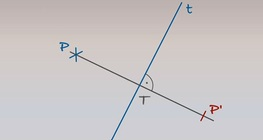#### The reflection of a point across an axis

Axis t and point P are given. Let’s plot the mirror image (point P’) of point P across axis t.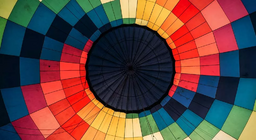#### The Cartesian and the polar coordinate system

Practise how to define a specific location using coordinate systems.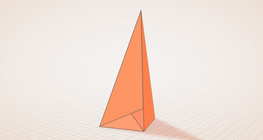#### Császár polyhedron

The Császár polyhedron is a nonconvex polyhedron with 14 triangular faces.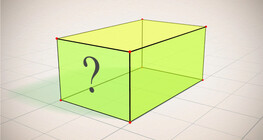#### Cuboid (exercises)

Edges, diagonals and faces of a cuboid can be identified by its vertices.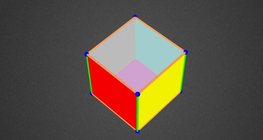#### Colouring a cube

Colouring the vertices, edges and faces of a given cube according to the criteria...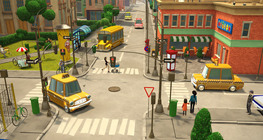#### Junction

Entertaining and spectacular animation to practise orientation in space.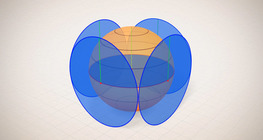#### Surface area of spheres (demonstration)

The surface of a sphere consists of the set of points which are all at the same distance...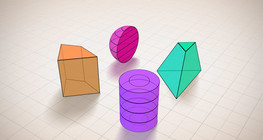#### Views

Pick the shape that is shown in different views below.

Added to your cart.